Example 83.2 Creating a One-Sided O’Brien-Fleming Design

This example demonstrates a group sequential design for a clinical study. A clinic is conducting a study on the effect of vitamin C supplements in treating flu symptoms. The study groups consist of patients in the clinic with their first sign of flu symptoms within the last 24 hours. These individuals are randomly assigned to either the control group, which receives the placebo pills, or the treatment group, which receives large doses of vitamin C supplements. At the end of a five-day period, the flu symptoms of each individual are recorded.

Suppose that from past experience, 60% of individuals experiencing flu symptoms have the symptoms disappeared within five days. The clinic wants to detect a 75% symptoms disappearance with a high probability in the trial. A test that compares the proportions directly is to specify a null hypothesis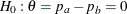with a Type I error probability level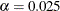, where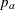and
are the proportions of symptoms’ disappearance in the treatment group and control group, respectively. A one-sided alternative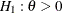is also specified with a power of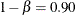at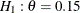.

For a one-sided fixed-sample design, the critical value for the standardized Z test statistic is given by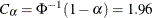. That is, at the end of study, if the test statistic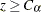, then the null hypothesis is rejected and the efficacy of vitamin C supplements is declared. Otherwise, the null hypothesis is not rejected and the effect of vitamin C supplements is not significant.

To achieve apower atfor a fixed-sample design, the information required is given by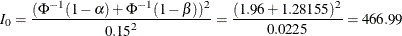With an equal sample size on the treatment and control groups,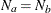, the sample size required for each group under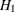is computed from the information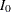: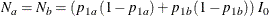where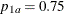and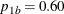are proportions in the treatment and control groups under. That is,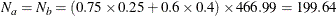Thus, 200 individuals are required for each group in the fixed-sample study. See the section Test for the Difference between Two Binomial Proportions for a detailed derivation of these required sample sizes.

Instead of a fixed-sample design for the trial, a group sequential design is used to stop the trial early for ethical concerns of possible harm or an unexpected strong efficacy outcome of the new drug. It can also save time and resources in the process. The following statements invoke the SEQDESIGN procedure and request a four-stage group sequential design that uses an O’Brien-Fleming method for normally distributed statistics. The design uses a one-sided alternative hypothesiswith early stopping to reject or accept.

ods graphics on;
proc seqdesign altref=0.15
;
OneSidedOBrienFleming: design nstages=4
method=obf
alt=upper   stop=both
alpha=0.025 beta=0.10
;
samplesize model=twosamplefreq(nullprop=0.6 test=prop);
ods output Boundary=Bnd_Prop;
run;
ods graphics off;

In a sequential design, a hypothesis can be rejected, accepted, or continued to the next time point at each interim stage. The STOP=BOTH option specifies early stopping to reject or accept the null hypothesis. The Design Information, Method Information, and Boundary Information tables are displayed by default.

The Design Information table in Output 83.2.1 displays design specifications and derived statistics such as power and maximum information. With a specified alternative reference, ALTREF=0.15, the maximum information
is derived.

The SEQDESIGN Procedure
Design: OneSidedOBrienFleming

Statistic Distribution Normal
Boundary Scale Standardized Z
Alternative Hypothesis Upper
Early Stop Accept/Reject Null
Method O'Brien-Fleming
Boundary Key Both
Alternative Reference 0.15
Number of Stages 4
Alpha 0.025
Beta 0.1
Power 0.9
Max Information (Percent of Fixed Sample) 107.6741
Max Information 502.8343
Null Ref ASN (Percent of Fixed Sample) 61.12891
Alt Ref ASN (Percent of Fixed Sample) 75.89782

The Max Information (Percent Fixed-Sample) is the ratio in percentage between the maximum information for the group sequential design and the information required for a corresponding fixed-sample design: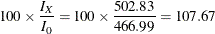That is, if the group sequential trial does not stop at any interim stages, the information needed is 7.67% more than is needed for the corresponding fixed-sample design. For a two-sample test for binomial proportions, the information is proportional to the sample size. Thus, 7.67% more observations are needed for the group sequential trial.

The Null Ref ASN (Percent Fixed-Sample) is the ratio in percentage between the expected sample size required under the null hypothesis for the group sequential design and the sample size required for the corresponding fixed-sample design. With a ratio of 61.1%, the expected sample size for the group sequential trial under the null hypothesis is 61.1% of the sample size in the corresponding fixed-sample design.

Similarly, the Alt Ref ASN (Percent Fixed-Sample) is the ratio in percentage between the expected sample size required under the alternative hypothesis for the group sequential design and the sample size required for the corresponding fixed-sample design. With a ratio of 75.9%, the expected sample size for the group sequential trial under the alternative hypothesis is 75.9% of the sample size in the corresponding fixed-sample design.

For a one-sided design with an upper alternative and early stopping to reject or accept the null hypothesis, upperandboundaries are created. The Method Information table in Output 83.2.2 displays the Type I error probability, the Type II error probability, and the derived drift parameter. The drift parameter is the standardized reference improvement between the alternative and null hypotheses at the final stage. It is also the standardized alternative reference at the final stage if the null reference is zero.

Output 83.2.2: Method Information

Method Information
Boundary Method Alpha Beta Unified Family Alternative
Reference
Drift
Rho Tau C
Upper Alpha O'Brien-Fleming 0.02500 . 0.5 0 1.9784 0.15 3.363595
Upper Beta O'Brien-Fleming . 0.10000 0.5 0 1.3852 0.15 3.363595

With the METHOD=OBF option, the O’Brien-Fleming method is used for each boundary. The O’Brien-Fleming method is one of the unified family methods, and the Method Information table displays the corresponding parameter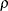in the unified family method. The table also displays the critical values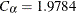for theboundary and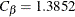for theboundary. These critical values are used to create the boundary values.

The Boundary Information table in Output 83.2.3 displays information level, alternative reference, and boundary values at each stage. By default (or equivalently if you specify BOUNDARYSCALE=STDZ), the alternative references and boundary values are displayed with the standardized Z statistic scale. The resulting standardized alternative reference at stage k is given by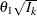, where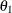is the alternative reference and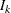is the information level at stage k,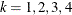.

Output 83.2.3: Boundary Information

Boundary Information (Standardized Z Scale)
Null Reference = 0
_Stage_   Alternative Boundary Values
Information Level Reference Upper
Proportion Actual N Upper Beta Alpha
1 0.2500 125.7086 107.4808 1.68180 -1.08860 3.95679
2 0.5000 251.4171 214.9617 2.37842 0.41946 2.79788
3 0.7500 377.1257 322.4425 2.91296 1.31347 2.28446
4 1.0000 502.8343 429.9233 3.36360 1.97840 1.97840

By default (or equivalently if you specify INFO=EQUAL), equally spaced information levels are used. An information proportion is the proportion of maximum information available at each stage. With the derived maximum information, the actual information level at each stage is also displayed. With the SAMPLESIZE statement, the required sample size N is also displayed under the heading Information Level.

At each interim stage, if the standardized Z test statistic is larger than or equal to the corresponding upperboundary, then the hypothesis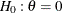is rejected. If the test statistic is less than the corresponding upperboundary, then the trial is stopped and the hypothesisis accepted. Otherwise, the process continues to the next stage. At the final stage, stage 4, the trial stops and the hypothesisis rejected if the standardized Z statistic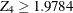. Otherwise, the trial is accepted.

The ODS OUTPUT statement with the BOUNDARY=BND_PROP option creates an output data set that contains the resulting boundary information. After the actual data from the clinical trial are collected and analyzed at each stage with a procedure such as PROC GENMOD, the SEQTEST procedure is used to test the resulting statistics at stage 1 with the boundary information stored in the BOUND_PROP data set.

With ODS Graphics enabled, a detailed boundary plot with the rejection and acceptance regions is displayed, as shown in Output 83.2.4.

Output 83.2.4: Boundary Plot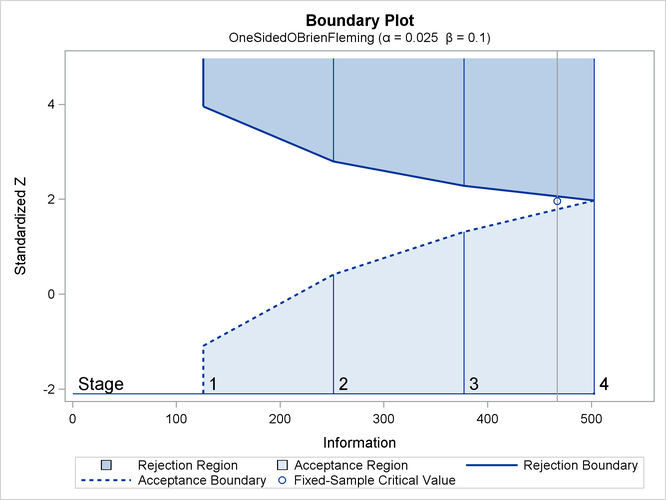The horizontal axis indicates the information levels for the design. The stages are indicated by vertical lines with accompanying stage numbers. If at any stage a test statistic is in a rejection region, the trial stops and the hypothesis is rejected. If a test statistic is in an acceptance region, then the trial also stops and the hypothesis is accepted. If the statistic is not in a rejection region or an acceptance region, the trial continues to the next stage. The boundary plot also displays the information level and the critical value for the corresponding fixed-sample design.

The SEQDESIGN procedure derives the drift parameter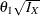, whereis the alternative reference andis the maximum information. If eitheroris specified, the other can be derived. With the SAMPLESIZE statement, the maximum information is used to compute the required sample size for the study.

The Sample Size Summary table in Output 83.2.5 displays parameters for the sample size computation. With the MODEL=TWOSAMPLEFREQ( NULLPROP=0.6 TEST=PROP) option in the SAMPLESIZE statement, the total sample size in each group for testing the difference between two proportions is computed. By default (or equivalently if you specify REF=PROP in the MODEL=TWOSAMPLEFREQ option), the required sample sizes are computed under the alternative hypothesis. That is,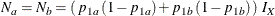where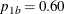and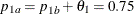are the proportions in the control and treatment groups, respectively, under the alternative hypothesis. See the section Test for the Difference between Two Binomial Proportions for a detailed description of these parameters.

Output 83.2.5: Sample Size Summary

Sample Size Summary
Test Two-Sample Proportions
Null Proportion 0.6
Proportion (Group A) 0.75
Test Statistic Z for Proportion
Reference Proportions Alt Ref
Max Sample Size 429.9233
Expected Sample Size (Null Ref) 244.0768
Expected Sample Size (Alt Ref) 303.0464

The Sample Sizes (N) table in Output 83.2.6 displays the required sample sizes at each stage, in both fractional and integer numbers. The derived fractional sample sizes are under the heading Fractional N. These sample sizes are rounded up to integers under the heading Ceiling N. In practice, integer sample sizes are used, and the resulting information levels increase slightly. Thus, 54, 108, 162, and 215 individuals are needed in each group for the four stages, respectively.

Output 83.2.6: Derived Sample Sizes

Sample Sizes (N)
Two-Sample Z Test for Proportion Difference
_Stage_ Fractional N Ceiling N
N N(Grp 1) N(Grp 2) Information N N(Grp 1) N(Grp 2) Information
1 107.48 53.74 53.74 125.7 108 54 54 126.3
2 214.96 107.48 107.48 251.4 216 108 108 252.6
3 322.44 161.22 161.22 377.1 324 162 162 378.9
4 429.92 214.96 214.96 502.8 430 215 215 502.9